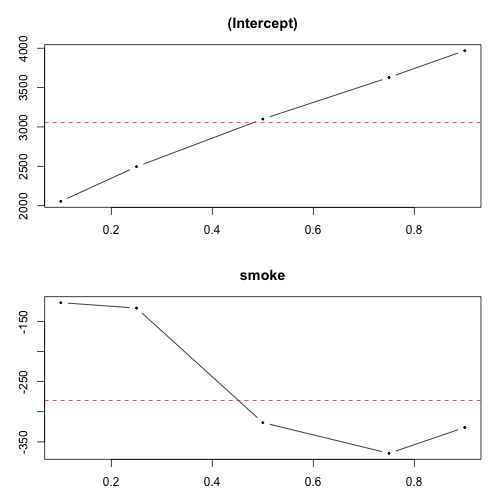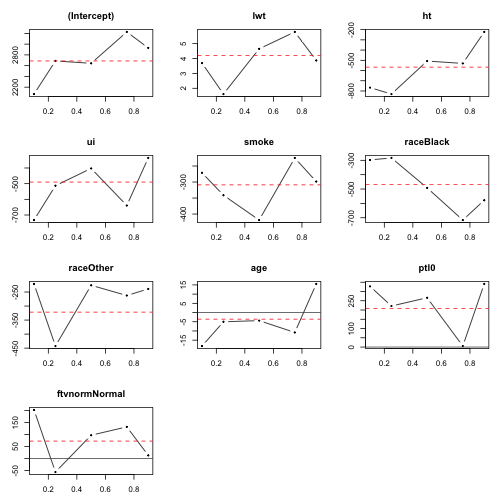# Quantile regression, including median regression

``````## Settings for RMarkdown http://yihui.name/knitr/options#chunk_options
opts_chunk\$set(comment = "", warning = FALSE, message = FALSE, tidy = FALSE,
echo = TRUE, fig.width = 7, fig.height = 7)
options(width = 116, scipen = 10)
``````

## References

Normality and constant variance assumptions are not necessary.

It is applicable to situations in which treatment/exposure response is dependent on the baseline outcome value. In linear regression, depending on the values of predictors, the predicted mean will shift along with the distribution of the outcome values.

``````library(gdata)

## Data available online: http://www.umass.edu/statdata/statdata/data/index.html
## Cases 10 and 39 needs fix to make them identical to Dr. Orav's dataset
lbw[c(10,39),"BWT"] <- c(2655,3035)
names(lbw) <- tolower(names(lbw))

## Recoding
library(car)
lbw <- within(lbw, {
## race relabeling
race <- factor(race, levels = 1:3, labels = c("White","Black","Other"))

## ftv (frequency of visit) relabeling
ftvnorm <- cut(ftv, breaks = c(-Inf, 0, 2, Inf), labels = c("Zero","Normal","High"))
ftvnorm <- car::recode(ftvnorm, 'c("Zero","High") = "Abnormal"')
ftvnorm <- relevel(ftvnorm, ref = "Abnormal")

## ptl0
ptl0 <- as.numeric(ptl == 0)
})
``````

## Create linear models

There is only one way to calculate the estimates and standard errors.

``````## Smoke only model
lm.bwt.by.smoke <- lm(bwt ~ smoke, data = lbw)
summary(lm.bwt.by.smoke)
``````
``````
Call:
lm(formula = bwt ~ smoke, data = lbw)

Residuals:
Min     1Q Median     3Q    Max
-2064   -478     35    545   1935

Coefficients:
Estimate Std. Error t value Pr(>|t|)
(Intercept)   3055.0       66.9   45.64   <2e-16 ***
smoke         -281.4      107.0   -2.63   0.0092 **
---
Signif. codes:  0 '***' 0.001 '**' 0.01 '*' 0.05 '.' 0.1 ' ' 1

Residual standard error: 718 on 187 degrees of freedom
Multiple R-squared: 0.0357, Adjusted R-squared: 0.0305
F-statistic: 6.92 on 1 and 187 DF,  p-value: 0.00923

``````
``````## Full model
lm.bwt.by.full <- lm(bwt ~ lwt + ht + ui + smoke + race + age + ptl0 + ftvnorm, data = lbw)
summary(lm.bwt.by.full)
``````
``````
Call:
lm(formula = bwt ~ lwt + ht + ui + smoke + race + age + ptl0 +
ftvnorm, data = lbw)

Residuals:
Min      1Q  Median      3Q     Max
-1897.8  -455.8    36.1   469.3  1668.5

Coefficients:
Estimate Std. Error t value        Pr(>|t|)
(Intercept)    2687.83     344.74    7.80 0.0000000000005 ***
lwt               4.19       1.71    2.45         0.01539 *
ht             -567.10     200.54   -2.83         0.00522 **
ui             -489.92     136.85   -3.58         0.00044 ***
smoke          -308.81     108.93   -2.83         0.00511 **
raceBlack      -469.25     149.50   -3.14         0.00199 **
raceOther      -321.85     117.20   -2.75         0.00664 **
age              -3.62       9.60   -0.38         0.70637
ptl0            208.63     136.12    1.53         0.12712
ftvnormNormal    72.25     102.35    0.71         0.48118
---
Signif. codes:  0 '***' 0.001 '**' 0.01 '*' 0.05 '.' 0.1 ' ' 1

Residual standard error: 646 on 179 degrees of freedom
Multiple R-squared: 0.253,  Adjusted R-squared: 0.215
F-statistic: 6.73 on 9 and 179 DF,  p-value: 0.0000000269

``````

## Create quantile regression models

The detrimental effect of smoking on birthweight is more prominent in the larger end of the distribution. The estimated effect is -119 at the 10th percentile, but -326 at the 90th percentile. In the linear model, the whole distribution is estimated to be lower by the value at red horizontal line.

Smoking only model

``````library(quantreg)
## Smoke only model
rq.bwt.by.smoke <- rq(bwt ~ smoke, tau = c(0.1, 0.25, 0.5, 0.75, 0.9), data = lbw)
rq.bwt.by.smoke
``````
``````Call:
rq(formula = bwt ~ smoke, tau = c(0.1, 0.25, 0.5, 0.75, 0.9),
data = lbw)

Coefficients:
tau= 0.10 tau= 0.25 tau= 0.50 tau= 0.75 tau= 0.90
(Intercept)      2055      2495      3100      3629      3969
smoke            -119      -128      -318      -369      -326

Degrees of freedom: 189 total; 187 residual
``````
``````plot(rq.bwt.by.smoke)
``````Full model

``````## Full model
rq.bwt.by.full <- rq(bwt ~ lwt + ht + ui + smoke + race + age + ptl0 + ftvnorm,
tau = c(0.1, 0.25, 0.5, 0.75, 0.9), data = lbw)
rq.bwt.by.full
``````
``````Call:
rq(formula = bwt ~ lwt + ht + ui + smoke + race + age + ptl0 +
ftvnorm, tau = c(0.1, 0.25, 0.5, 0.75, 0.9), data = lbw)

Coefficients:
tau= 0.10 tau= 0.25 tau= 0.50 tau= 0.75 tau= 0.90
(Intercept)    2072.428  2687.856  2643.356  3226.422  2929.915
lwt               3.687     1.613     4.645     5.778     3.868
ht             -767.242  -830.130  -507.308  -530.481  -222.632
ui             -732.185  -512.942  -402.877  -639.553  -335.962
smoke          -271.245  -341.217  -418.761  -224.829  -298.547
raceBlack      -297.349  -283.511  -492.038  -716.165  -578.132
raceOther      -221.192  -442.625  -225.958  -262.457  -238.981
age             -18.195    -5.026    -4.386   -10.808    15.519
ptl0            327.553   221.588   266.015     5.374   340.708
ftvnormNormal   202.233   -56.831    96.749   131.575    12.670

Degrees of freedom: 189 total; 179 residual
``````
``````plot(rq.bwt.by.full)
``````## Standard errors

No established standard exist for calculation of standard erros in quantile regression.

References:

help for summary.rq

``````      se: specifies the method used to compute standard standard
errors.  There are currently five available methods:

1. ‘"rank"’ which produces confidence intervals for the
estimated parameters by inverting a rank test as
described in Koenker (1994).  The default option assumes
that the errors are iid, while the option iid = FALSE
implements the proposal of Koenker Machado (1999).  See
the documentation for ‘rq.fit.br’ for additional
arguments.

2. ‘"iid"’ which presumes that the errors are iid and
computes an estimate of the asymptotic covariance matrix
as in KB(1978).

3. ‘"nid"’ which presumes local (in ‘tau’) linearity (in
‘x’) of the the conditional quantile functions and
computes a Huber sandwich estimate using a local estimate
of the sparsity.

4. ‘"ker"’ which uses a kernel estimate of the sandwich as
proposed by Powell(1990).

5. ‘"boot"’ which implements one of several possible
bootstrapping alternatives for estimating standard
errors.

If ‘se = NULL’ (the default) and ‘covariance = FALSE’ then
the "rank" method is used, otherwise the "nid" method is
used.
``````

The fifth method, boot (bootstrap) results in different standard error everytime.

``````rq.bwt.by.smoke <- rq(bwt ~ smoke, tau = c(0.5), data = lbw)

summary(rq.bwt.by.smoke, se = "rank")
``````
``````
Call: rq(formula = bwt ~ smoke, tau = c(0.5), data = lbw)

tau:  0.5

Coefficients:
coefficients lower bd upper bd
(Intercept) 3100.00      2835.64  3337.72
smoke       -318.00      -611.03   -98.16
``````
``````summary(rq.bwt.by.smoke, se = "iid")
``````
``````
Call: rq(formula = bwt ~ smoke, tau = c(0.5), data = lbw)

tau:  0.5

Coefficients:
Value      Std. Error t value    Pr(>|t|)
(Intercept) 3100.00000  103.17389   30.04636    0.00000
smoke       -318.00000  164.88640   -1.92860    0.05529
``````
``````summary(rq.bwt.by.smoke, se = "nid")
``````
``````
Call: rq(formula = bwt ~ smoke, tau = c(0.5), data = lbw)

tau:  0.5

Coefficients:
Value      Std. Error t value    Pr(>|t|)
(Intercept) 3100.00000   93.91325   33.00919    0.00000
smoke       -318.00000  146.10502   -2.17652    0.03077
``````
``````summary(rq.bwt.by.smoke, se = "ker")
``````
``````
Call: rq(formula = bwt ~ smoke, tau = c(0.5), data = lbw)

tau:  0.5

Coefficients:
Value      Std. Error t value    Pr(>|t|)
(Intercept) 3100.00000  116.10295   26.70044    0.00000
smoke       -318.00000  176.01254   -1.80669    0.07242
``````
``````summary(rq.bwt.by.smoke, se = "boot")
``````
``````
Call: rq(formula = bwt ~ smoke, tau = c(0.5), data = lbw)

tau:  0.5

Coefficients:
Value      Std. Error t value    Pr(>|t|)
(Intercept) 3100.00000   88.01510   35.22123    0.00000
smoke       -318.00000  168.57334   -1.88642    0.06079
``````

In the bootstrap method, 200 replication with the xy method is the default. In SAS, mcmb is the default.

help for boot.rq

``````bsmethod: The method to be employed.  There are (as yet) five options:
method = "xy" uses the xy-pair method, and method = "pwy"
uses the method of Parzen, Wei and Ying (1994) method =
"mcmb" uses the Markov chain marginal bootstrap of He and Hu
(2002) and Kocherginsky, He and Mu (2003).  The fourth method
= "wxy" uses the generalized bootstrap of Bose and Chatterjee
and Imbens (2003).  The fifth method "wild" uses the wild
bootstrap method proposed by Feng, He and Hu (2011).
``````
``````summary(rq.bwt.by.smoke, se = "boot", R = 200, bsmethod = "xy")
``````
``````
Call: rq(formula = bwt ~ smoke, tau = c(0.5), data = lbw)

tau:  0.5

Coefficients:
Value      Std. Error t value    Pr(>|t|)
(Intercept) 3100.00000   91.77545   33.77810    0.00000
smoke       -318.00000  167.40859   -1.89954    0.05903
``````
``````summary(rq.bwt.by.smoke, se = "boot", R = 200, bsmethod = "pwy")
``````
``````
Call: rq(formula = bwt ~ smoke, tau = c(0.5), data = lbw)

tau:  0.5

Coefficients:
Value      Std. Error t value    Pr(>|t|)
(Intercept) 3100.00000   88.01045   35.22309    0.00000
smoke       -318.00000  170.18255   -1.86858    0.06324
``````
``````summary(rq.bwt.by.smoke, se = "boot", R = 200, bsmethod = "mcmb")
``````
``````
Call: rq(formula = bwt ~ smoke, tau = c(0.5), data = lbw)

tau:  0.5

Coefficients:
Value    Std. Error t value  Pr(>|t|)
(Intercept) 3100.000   92.681     33.448    0.000
smoke       -318.000  168.713     -1.885    0.061
``````
``````summary(rq.bwt.by.smoke, se = "boot", R = 200, bsmethod = "wxy")
``````
``````
Call: rq(formula = bwt ~ smoke, tau = c(0.5), data = lbw)

tau:  0.5

Coefficients:
Value      Std. Error t value    Pr(>|t|)
(Intercept) 3100.00000   90.12303   34.39742    0.00000
smoke       -318.00000  166.68975   -1.90774    0.05796
``````
``````summary(rq.bwt.by.smoke, se = "boot", R = 200, bsmethod = "wild")
``````
``````
Call: rq(formula = bwt ~ smoke, tau = c(0.5), data = lbw)

tau:  0.5

Coefficients:
Value      Std. Error t value    Pr(>|t|)
(Intercept) 3100.00000   88.45409   35.04643    0.00000
smoke       -318.00000  166.95339   -1.90472    0.05835
``````

quantreg::FAQ() function gives FAQs

``````6.  [Confidence Intervals]  "Why does summary(rq(...)) sometimes produce a
table with upper and lower confidence limits, and sometimes a table with standard
errors?"

There are several methods for computing standard errors and confidence limits.
By default on small problems (n < 1001)  summary uses the rank inversion method,
which produces (potentially asymmetric) confidence intervals,while for larger
problems the default is to estimate the asymptotic covariance matrix and
report standard errors of the parameter estimates.
``````